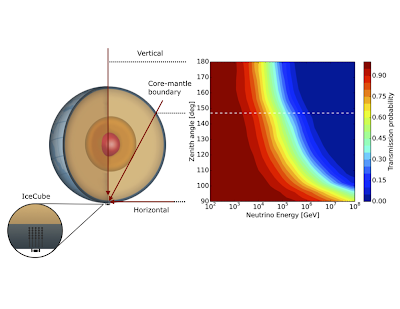Friday, December 8, 2017

Stopping a neutrino beam; measuring their interaction cross-section

Neutrinos are popularly known as the particles that go through anything and everything.  Neutrinos from beta decay can escape from the best shielded nuclear reactor, and neutrinos from nuclear fusion escape from the center of the sun.  Neutrinos interact only via the weak interaction, which is indeed weak.  But, that doesn't mean that they can go through anything - the IceCube Neutrino Observatory recently demonstrated experimentally that it is possible to stop a beam of neutrinos, in a paper published in Nature (also freely available on the arXiv).

To do this, IceCube used two tricks.

First, it use extremely energetic neutrinos, with energies above 1 TeV (1 tera-electron volt, or 1012 electron Volts), extending up to 1 PeV (1 peta-electron volt, or 1015 eV), millions of times more energetic than neutrinos from nuclear fusion or radioactive ion decay.  The cross-section (probability) for neutrinos to interact rises with energy (linearly at first, then moderated to scale roughly as Energy0.3.  So, at an energy of 30 TeV (the rough mid-point of the measurement) the cross-section is several million times higher than it is for neutrinos from radioactive decay.  Of course, there aren't that many neutrinos this energetic, but, at 1 cubic kilometer in volume,  IceCube is big enough to collect a good sample.  The analysis used 10, 784 energetic muons from neutrinos that passed through at least some of the Earth.Second, it used a very thick absorber - the Earth.  With this, the measurement was quite simple.   It Compared to a baseline of near-horizontal neutrinos that traversed only a relatively small amount of matter, energetic near-vertical neutrinos were absorbed going through the Earth.  The figure above shows the predicted transmission probability (= 1 - absorption probability), as a function of neutrino energy and zenith angle; the latter shows how much Earth matter was traversed.

There are of course many complications - experimental uncertainties on the neutrino energy, neutral current interactions, where a neutrino may emerge from the Earth with a lower energy than it entered, modelling the material within the Earth, etc., but the result clearly showed that neutrinos are absorbed at about the expected rate.  More precisely, the best-fit cross-section was. 1.3 +/- 0.5 times the predictions of the Standard model where I have combined the statistical and systematic uncertainty.  It was not trivial to find a good definition for the neutrino energy range for which this measurement applies, because different methods give somewhat different energy ranges, but we settled on a method that returned a range from 6.3 TeV to 980 TeV.  For comparison, the highest energy measurements at an accelerator laboratory only reached 0.37 TeV - our measurement reaches order of magnitude higher energies than than.  The figure below puts this in perspective, comparing our measurement with the previous accelerator work.  The cross-sections (y axis) are divided by the neutrino energy so that everything fits on the graph better; otherwise, it would span many orders of magnitude.

I have to mention that this was the dissertation work of my (now graduated) graduate student, Sandra Miarecki.  Sandy had a very interesting preparation for graduate school - she was a career US Air Force Pilot, serving many roles, including as a test pilot, before retiring from the Air Force and coming to graduate school in Berkeley.   After graduate school, she became an Assistant professor at the US Air Force Academy.   The LBNL news center has a very nice article about her.

The Nature article also recieved a fair amount of press coverage.  I will just mention one article,  in Symmetry magazine, which goes into more detail about the analysis than other press writeups.